# 推荐系统论文回顾：神经协同过滤理解与实现# 矩阵分解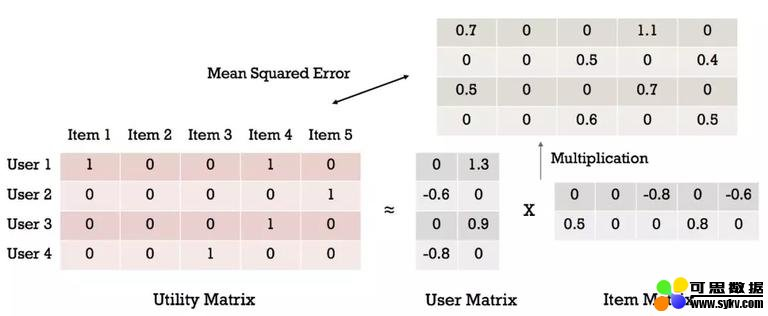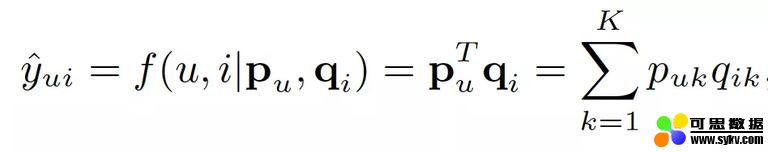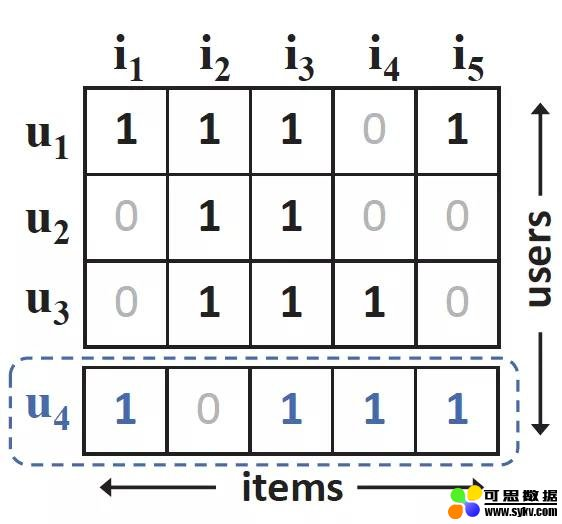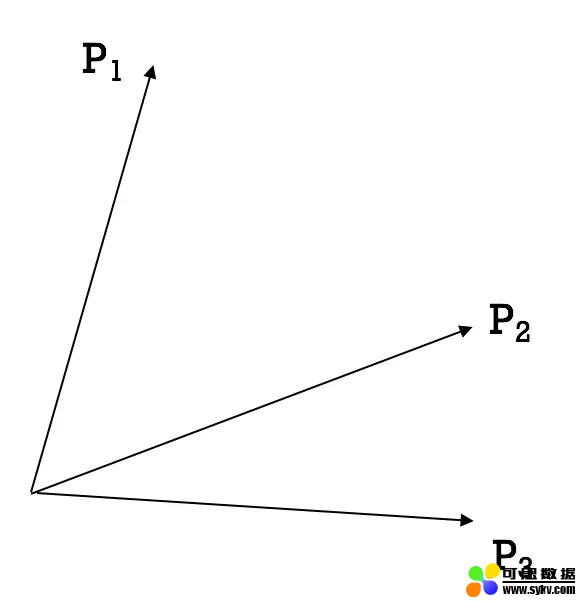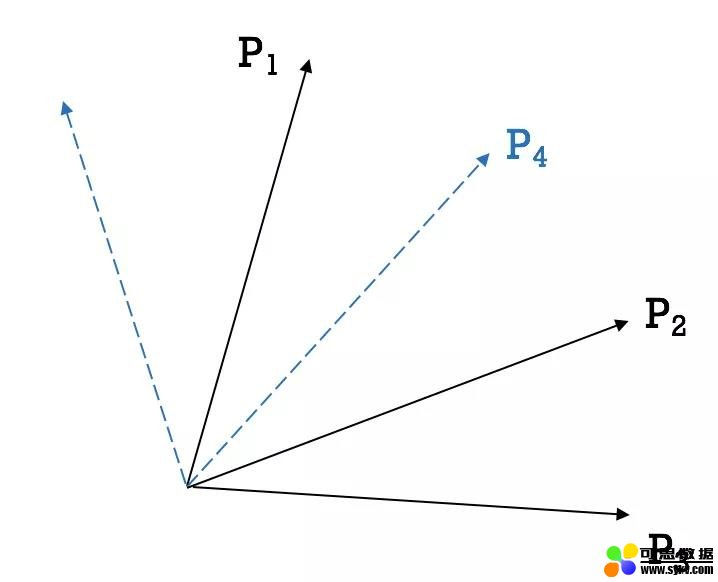# 神经协同过滤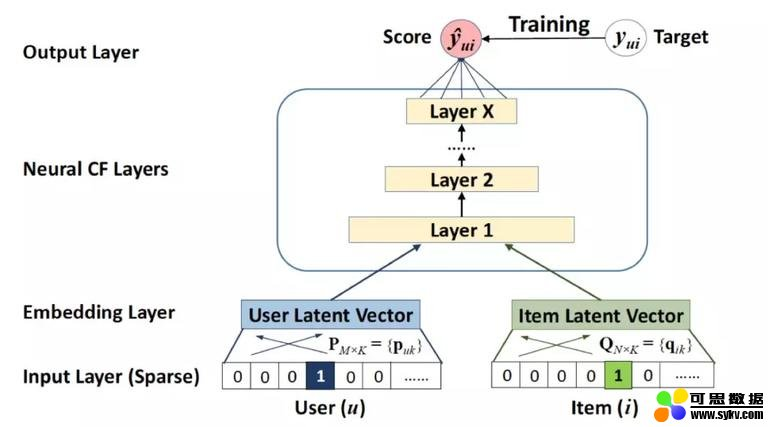• 本文地址：http://www.6aiq.com/article/1578837141466
• 本文版权归作者和AIQ共有，欢迎转载，但未经作者同意必须保留此段声明，且在文章页面明显位置给出
• 知乎专栏 点击关注

NCF 结构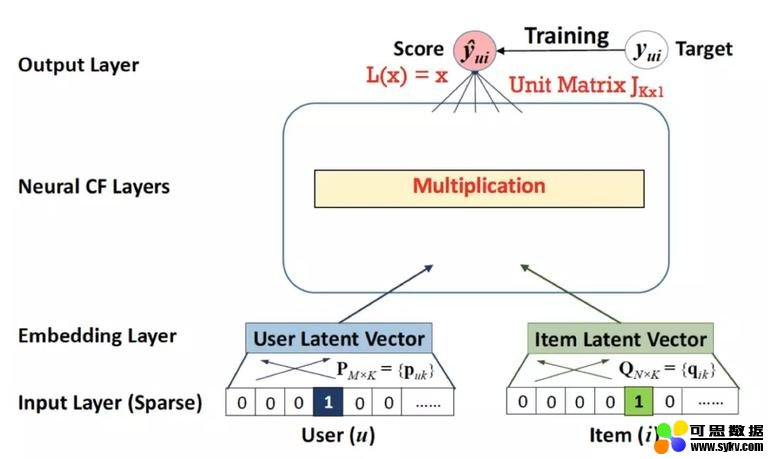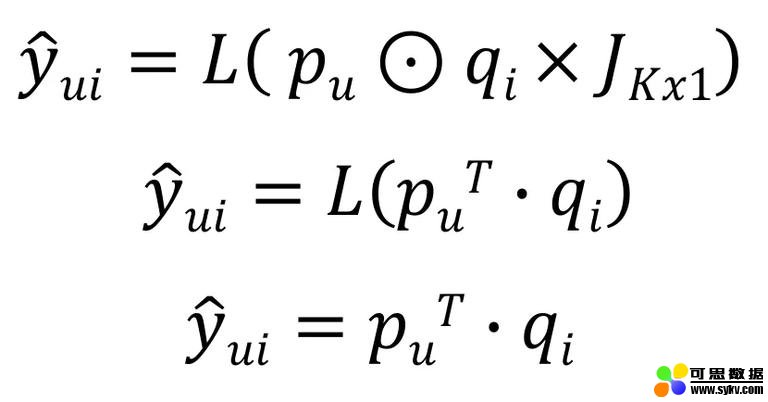# NeuMF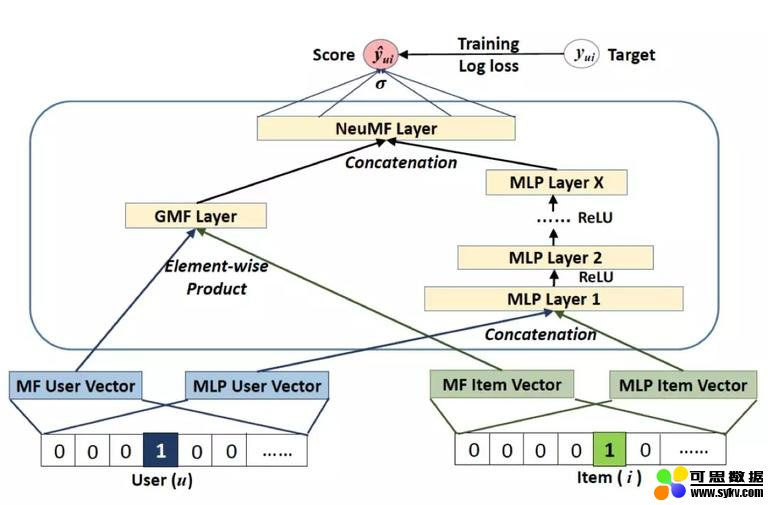GMF 和 MLP 模块的输出连接到 sigmoid 激活输出层。

# 性能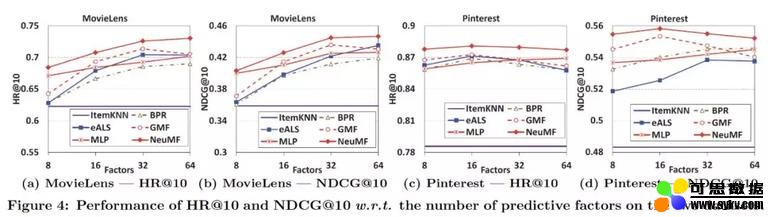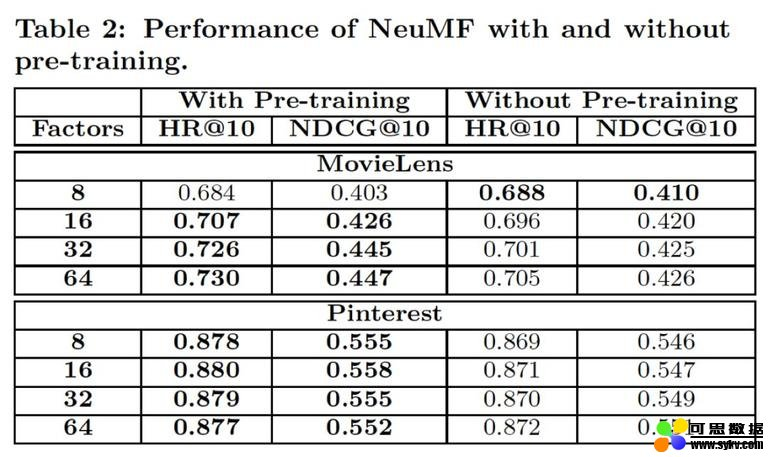# 实现

``````def __init__(self, config):
super(NeuMF, self).__init__()

#mf part
self.embedding_user_mf = torch.nn.Embedding(num_embeddings=self.num_users, embedding_dim=self.latent_dim_mf)
self.embedding_item_mf = torch.nn.Embedding(num_embeddings=self.num_items, embedding_dim=self.latent_dim_mf)

#mlp part
self.embedding_user_mlp = torch.nn.Embedding(num_embeddings=self.num_users, embedding_dim=self.latent_dim_mlp)
self.embedding_item_mlp = torch.nn.Embedding(num_embeddings=self.num_items, embedding_dim=self.latent_dim_mlp)

self.fc_layers = torch.nn.ModuleList()
for idx, (in_size, out_size) in enumerate(zip(config['layers'][:-1], config['layers'][1:])):
self.fc_layers.append(torch.nn.Linear(in_size, out_size))

self.logits = torch.nn.Linear(in_features=config['layers'][-1] + config['latent_dim_mf'] , out_features=1)
self.sigmoid = torch.nn.Sigmoid()
``````

``````def forward(self, user_indices, item_indices, titles):
user_embedding_mlp = self.embedding_user_mlp(user_indices)
item_embedding_mlp = self.embedding_item_mlp(item_indices)
user_embedding_mf = self.embedding_user_mf(user_indices)
item_embedding_mf = self.embedding_item_mf(item_indices)

#### mf part
mf_vector =torch.mul(user_embedding_mf, item_embedding_mf)
mf_vector = torch.nn.Dropout(self.config.dropout_rate_mf)(mf_vector)

#### mlp part
mlp_vector = torch.cat([user_embedding_mlp, item_embedding_mlp], dim=-1)  # the concat latent vector

for idx, _ in enumerate(range(len(self.fc_layers))):
mlp_vector = self.fc_layers[idx](mlp_vector "idx")
mlp_vector = torch.nn.ReLU()(mlp_vector)
mlp_vector = torch.nn.Dropout(self.config.dropout_rate_mlp)(mlp_vector)

vector = torch.cat([mlp_vector, mf_vector], dim=-1)
logits = self.logits(vector)
output = self.sigmoid(logits)
return output

``````

`forward()`函数相当简单。我们只是让用户和物品索引在定义的网络中流动。特别要注意的是，我在 GMP 和 MLP 模块的末尾添加了 Dropout 层，因为我发现这有助于网络的正则化和性能的提高。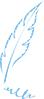—END—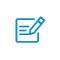扫码入群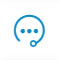咨询反馈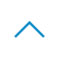返回顶部